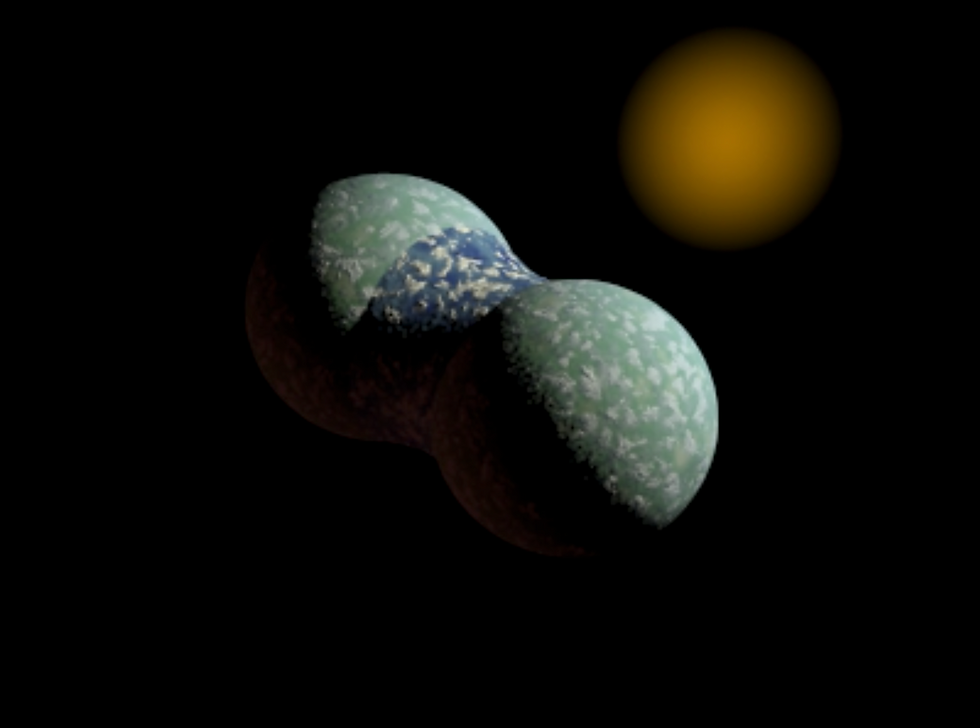top of page
Search

# What shape is the ocean on a cubical planet?

Here's a repost of something I wrote almost a decade ago, wondering what shape an ocean would take on a non-spherical planet. I do hope the flat-earthers enjoy this.

Cut to the chase - Clearly, they are circular!The question is, however, how do you calculate this?

The first step is to realise that the surface of a liquid is an equipotential surface. This means nothing more than the value of the potential energy over the surface is the same. This is easy to think about if you remember your classical mechanics as if there were differences in the potential energies in the water, so one bit of water was higher than the other, then that potential can be converted to kinetic as the water flows downwards. When could all this be static? When the surface of the water is at the same potential, so no one bit is "higher" (in terms of potential energy) than another.

So, to work out the shape of the ocean, we just need to calculate surfaces of the same potential energy. But how do we do that for our cubical world?

In the following, I've made a few simplifying assumptions. I assume my planet is not rotating (that adds a further set of energy terms) and that I can ignore the mass of the water (so ignore its potential energy). All I need to do is work out the potential energy of the cubical planet!

Many will remember from school that the potential energy of a point-like mass is given bywhere M is the mass, G is Newton's gravitational constant and r is the distance away from the mass.

One thing that often confuddles students is that this expression diverges as r goes to zero. But what's the potential of an extended mass distribution? Well, then we need to add up all the little mass bits to give the total potential which, when we have a continuous mass distribution, we can represent as an integral. So the potential now looks like thisLet's not worry about the details, but those in the know will know that such an integral can be done quite efficiently as a convolution using Fourier transforms. If you are not in the know, just put it down to mathemagic.

Once we know the potential, we just plot up up the surface of the planet and the surface of the water (and equipotential) and voila. I choose to use povray for this.

Let's add a little more water. What do we get?Notice that the water bulges out significantly. So what would life be like on the cubical planet? Well, it should be clear that the corner of the cubes would be like mountains, and so if you lived in the centre of a cube face, you would be able to stand upright, but as you walks towards the edge you would feel the orientation of the gravitational field changing. As you move away from the centre, it would feel that the climb was getting steeper and steeper, event though you are on an apparently flat plane!

But remember that the surfaces of the water are equipotentials, so you can happily sail over the ocean with little effort. In fact with a little more water, we can sail right around the planet. The view would be cool!But what about other planets? What would the ocean be like on a dumbbell planet? EasyAn uneven dumbbellSlab planetThe possibilities are endless :) I'll play some more with this in the future.

In closing, I just want to note that I didn't design the planetary textures in povray, but I have lost the reference to where I got it from (although it is called Cheap World). Thanks to the original author!